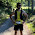## Sunday, January 18, 2015

### Averages are not good enough (F#)

Let's (no pun intended) look at a set of response times of a web service.

People like having a single number to summarize a piece of data. The average is the most popular candidate. The average is calculated by dividing the sum of the input elements by the number of input elements.

The average is a measure of centre which is fragile to outliers; one or two odd irregular values might skew the outcome. The median on the other hand is always representative of the centre, not just when the data distribution is symmetric. The median is determined by sorting the input elements and picking the one in the middle.

Both measures are terrible at showing how the data is distributed though. The average and median response time might look fair, but maybe there are a few outliers which are giving a few customers a bad time.

Instead of reducing our input down to a single number, it might be better to extract a table that displays the frequency of various outcomes in the input.

Now this is more useful; we can clearly see that the data is not distributed equally and there are a few outliers in our response times we need to investigate further.

This table takes up quite a bit of ink though. What if we want to get rid of the table, but maintain a feel for the distribution of the data?

The standard deviation measures the amount of variation from the average. A low standard deviation means that the data points are very close to the mean. A high standard deviation indicates that the data points are spread out over a large range of values.
It is calculated by taking the square root of the average of the squared differences of the values from their average value.

The standard deviation is even more useful when you put the average at the centre of a graph, lay out the input elements according their deviation of the average and see a bell graph drawn. This means that we can use the empirical 68-95-99.7 rule to get a feel of how the data is distributed.
In statistics, the so-called 68–95–99.7 rule is a shorthand used to remember the percentage of values that lie within in a band around the mean in a normal distribution with a width of one, two and three standard deviations, respectively; more accurately, 68.27%, 95.45% and 99.73% of the values lie within one, two and three standard deviations of the mean, respectively.

For our set of response times, this means that 68.27% of the response times lies within the 24.8 and 66.4 range, 95.45% lies within the 4 and 87.2 range, while 99.73% lies within the -16.8 and 108 range.

When we calculate the standard deviation, we can put one extra number next to the average and derive from just two numbers how the data is distributed.

In conclusion, the mean and the median hide outliers. Looking at the frequency distribution gives you a more complete picture. If we insist on having less data to look at, the standard deviation and the 68–95–99.7 rule can compress the same complete picture into just two numbers.

1.Nice post; a good way to visualize this info is a boxplot, or box and whisker plot.

2.A couple of notes:

First, the calculation of the standard deviation differs for population and sample based values (for examples, check wikipedia). You are using the population standard deviation, while the sample standard deviation would probably be better in a real scenario (the change is simple, just subtract 1 of 'y' in your code).

Second, server response times are not normally distributed. The Log-Normal distribution is a lot more accurate when modeling server response times.

Third, a better single value to optimize would be something like the 95,98 or 99th percentile.

1.Good feedback, thanks!

3.Great article..... drowning it too much data is becoming more and more common and dealing with averages isn't the answer...... the majority of reporting only needs a meaning full summary figure that can be tracked and monitored and using SD could solve some of my issues.... much appreciated